## ErrorsWhen an error occurs in Python, you may see a chunk of red text like this.

This is very useful when creating programs as it tells us the exact line of the error (10), and its type (NameError).

However, a completed program should have code in place for when an unexpected error occurs – we call this exception handling.

## General Exception

In this example, Python will attempt to run the code indented beneath try.

If there are no errors then the code will stop just before except.

If an error does occur then the Exception code will be run.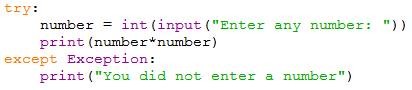If we enter a correct value then the program will execute normally:But if an error occurs (such as writing a string when an integer is expected) then the Exception code will run:You can add the else command to your code that will execute only if there are no errors: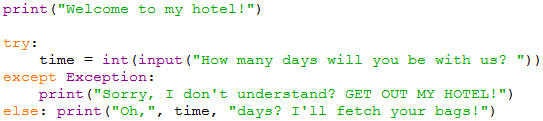If a valid number is entered then the else code will be printed: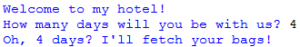If a code generating an error is entered then the except code will be printed:Create a program that asks the user to input their age.

Don't forget to use the int command.

Use try and except to print a message if a number is not inputted.

Example solution: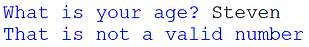## Specific Exceptions

The Exception command used in the section above is for any general error that occurs.

You can also use specific except commands for a variety of errors.

Below is a program with two different specific exception commands for one try statement:If a Value Error occurs, such as when the wrong data type is entered, then related code will be printed: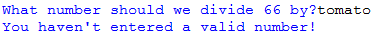Or if the user tries to divide by zero then a Zero Division Error will be triggered which prints a relevant response:Other types of exception can be found.

Create a program that asks the user to input a number and then divides this value by 999.

Create a Value Error and Zero Division Error exception and include an appropriate message in both.

Example solution for Zero Division: